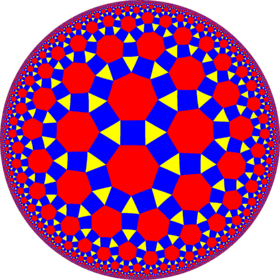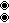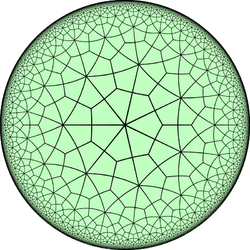# Rhombitriheptagonal tiling

Rhombitriheptagonal tilingPoincaré disk model of the hyperbolic plane
TypeHyperbolic uniform tiling
Vertex configuration3.4.7.4
Schläfli symbolrr{7,3} orWythoff symbol3 | 7 2
Coxeter diagramorSymmetry group[7,3], (*732)
DualDeltoidal triheptagonal tiling
PropertiesVertex-transitive

In geometry, the rhombitriheptagonal tiling is a semiregular tiling of the hyperbolic plane. At each vertex of the tiling there is one triangle and one heptagon, alternating between two squares. The tiling has Schläfli symbol rr{7, 3}. It can be seen as constructed as a rectified triheptagonal tiling, r{7,3}, as well as an expanded heptagonal tiling or expanded order-7 triangular tiling.

## Dual tiling

The dual tiling is called a deltoidal triheptagonal tiling, and consists of congruent kites. It is formed by overlaying an order-3 heptagonal tiling and an order-7 triangular tiling.From a Wythoff construction there are eight hyperbolic uniform tilings that can be based from the regular heptagonal tiling.

Drawing the tiles colored as red on the original faces, yellow at the original vertices, and blue along the original edges, there are 8 forms.

### Symmetry mutations

This tiling is topologically related as a part of sequence of cantellated polyhedra with vertex figure (3.4.n.4), and continues as tilings of the hyperbolic plane. These vertex-transitive figures have (*n32) reflectional symmetry.Wikimedia Commons has media related to Uniform tiling 3-4-7-4.